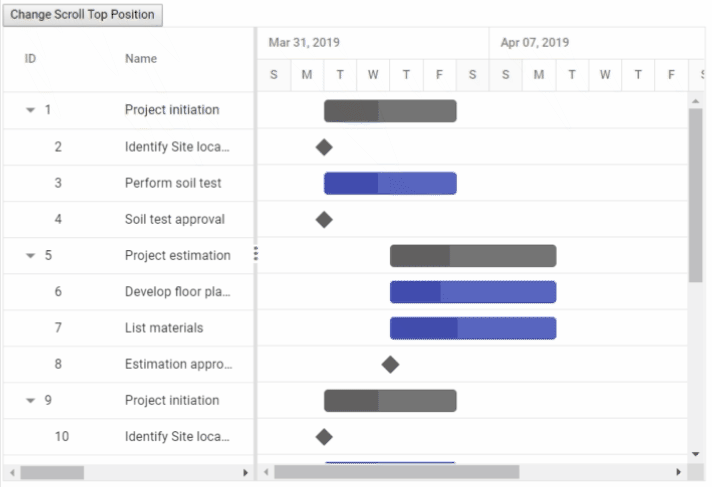Search results

# Set vertical scroll position

03 Mar 2021 / 4 minutes to read

In the Gantt Chart component, you can set the vertical scroller position dynamically by clicking the custom button using the `SetScrollTop` method.

``````@using Syncfusion.Blazor.Gantt
<button @onclick="SetScrollTop">Change Scroll Top Position</button>
<SfGantt @ref="Gantt" DataSource="@TaskCollection" Height="450px" Width="700px">
<GanttTaskFields Id="TaskId" Name="TaskName" StartDate="StartDate" EndDate="EndDate" Duration="Duration" Progress="Progress" Child="SubTasks">
</GanttTaskFields>
</SfGantt>
@code{
public SfGantt<TaskData> Gantt;
public void SetScrollTop()
{
this.Gantt.SetScrollTop(300);
}
public List<TaskData>
TaskCollection { get; set; }
protected override void OnInitialized()
{
this.TaskCollection = GetTaskCollection();
}

public class TaskData
{
public int TaskId { get; set; }
public string TaskName { get; set; }
public DateTime StartDate { get; set; }
public DateTime EndDate { get; set; }
public string Duration { get; set; }
public int Progress { get; set; }
public List<TaskData>
SubTasks { get; set; }
}

public static List <TaskData> GetTaskCollection() {
List <TaskData> Tasks = new List <TaskData> () {
new TaskData() {
TaskId = 1,
TaskName = "Project initiation",
StartDate = new DateTime(2019, 04, 02),
EndDate = new DateTime(2019, 04, 21),
SubTasks = (new List <TaskData> () {
new TaskData() {
TaskId = 2,
TaskName = "Identify Site location",
StartDate = new DateTime(2019, 04, 02),
Duration = "0",
Progress = 30,
},
new TaskData() {
TaskId = 3,
TaskName = "Perform soil test",
StartDate = new DateTime(2019, 04, 02),
Duration = "4",
Progress = 40,
},
new TaskData() {
TaskId = 4,
TaskName = "Soil test approval",
StartDate = new DateTime(2019, 04, 02),
Duration = "0",
Progress = 30
},
})
},
new TaskData() {
TaskId = 5,
TaskName = "Project estimation",
StartDate = new DateTime(2019, 04, 02),
EndDate = new DateTime(2019, 04, 21),
SubTasks = (new List <TaskData> () {
new TaskData() {
TaskId = 6,
TaskName = "Develop floor plan for estimation",
StartDate = new DateTime(2019, 04, 04),
Duration = "3",
Progress = 30,
},
new TaskData() {
TaskId = 7,
TaskName = "List materials",
StartDate = new DateTime(2019, 04, 04),
Duration = "3",
Progress = 40
},
new TaskData() {
TaskId = 8,
TaskName = "Estimation approval",
StartDate = new DateTime(2019, 04, 04),
Duration = "0",
Progress = 30,
}
})
},
new TaskData() {
TaskId = 9,
TaskName = "Project initiation",
StartDate = new DateTime(2019, 04, 02),
EndDate = new DateTime(2019, 04, 21),
SubTasks = (new List <TaskData> () {
new TaskData() {
TaskId = 10,
TaskName = "Identify Site location",
StartDate = new DateTime(2019, 04, 02),
Duration = "0",
Progress = 30,
},
new TaskData() {
TaskId = 11,
TaskName = "Perform soil test",
StartDate = new DateTime(2019, 04, 02),
Duration = "4",
Progress = 40,
},
new TaskData() {
TaskId = 12,
TaskName = "Soil test approval",
StartDate = new DateTime(2019, 04, 02),
Duration = "0",
Progress = 30
},
})
},
new TaskData() {
TaskId = 13,
TaskName = "Project estimation",
StartDate = new DateTime(2019, 04, 02),
EndDate = new DateTime(2019, 04, 21),
SubTasks = (new List <TaskData> () {
new TaskData() {
TaskId = 14,
TaskName = "Develop floor plan for estimation",
StartDate = new DateTime(2019, 04, 04),
Duration = "3",
Progress = 30,
},
new TaskData() {
TaskId = 15,
TaskName = "List materials",
StartDate = new DateTime(2019, 04, 04),
Duration = "3",
Progress = 40
},
new TaskData() {
TaskId = 16,
TaskName = "Estimation approval",
StartDate = new DateTime(2019, 04, 04),
Duration = "0",
Progress = 30,
}
})
},
new TaskData() {
TaskId = 17,
TaskName = "Project initiation",
StartDate = new DateTime(2019, 04, 02),
EndDate = new DateTime(2019, 04, 21),
SubTasks = (new List <TaskData> () {
new TaskData() {
TaskId = 18,
TaskName = "Identify Site location",
StartDate = new DateTime(2019, 04, 02),
Duration = "0",
Progress = 30,
},
new TaskData() {
TaskId = 19,
TaskName = "Perform soil test",
StartDate = new DateTime(2019, 04, 02),
Duration = "4",
Progress = 40,
},
new TaskData() {
TaskId = 20,
TaskName = "Soil test approval",
StartDate = new DateTime(2019, 04, 02),
Duration = "0",
Progress = 30
},
})
},
};

return Tasks;
}
}``````